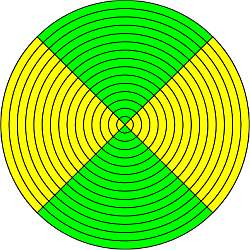# Symmetric Matrix Calculator

Instructions: Use this calculator to determine where a given matrix is symmetric or not, showing all the steps. All you have to do is to provide a matrix $$A$$ by typing its values below.

Modify, if needed, the size of the matrices by indicating the number of rows and the number of columns. Once you have the correct dimensions you want, you input the matrices (by typing the numbers and moving around the matrix using "TAB")

Number of Rows =    Number of Cols =

$$A$$ = \begin{bmatrix} & \\ & \end{bmatrix}

## More this symmetric matrix calculator

Symmetric matrices are special matrices that hold very neat properties. First of all, a symmetric matrix is a type of a square matrix with the property that its rows are exactly the same as its columns.

Another way of seeing that, a symmetric matrix is square matrix with the property that when you take its transpose, you get the exact original matrix.

Therefore, the shorthand definition is: A matrix $$A$$ is symmetric when $$A^T = A$$.### How do you find out if a matrix is symmetric?

Checking whether or not a matrix is symmetric is a relatively simple operation, at least compared with other more complicated and involved matrix procedures, such as matrix multiplications, or finding the inverse of a matrix.

You should follow the simple steps shown below in order to determine if a matrix is symmetric.

Step 1: Get the original matrix given $$A$$ and compute its transpose matrix

Step 2: Once you have computed the transpose matrix $$A^T$$, now compare it with the original matrix, term by term.

Step 3: If all the elements of the transpose matrix coincide with the elements of the original matrix, then the matrix is symmetric.

### What is the formula of symmetry of a matrix

The formula of symmetry of a matrix is $$A^T = A$$, which of of times is written in terms of the components, as $$A^T_{ij} = A_{ij}$$. Another way to express the same is using the formula of symmetry is $$A{ij} = A_{ji}$$### Symmetric matrix example

The matrix below gives you an example of a symmetric matrix:

$\begin{bmatrix} 1 & 2 & 3 \\ 2 & 4 & 5 \\ 3 & 4 & 1 \end{bmatrix}$

How can you tell it is symmetric? Well, just compute its transpose by getting the columns of the original matrix and place them as the rows of the transpose. And you will see that in this case, $$A^T = A$$. So it is symmetric.